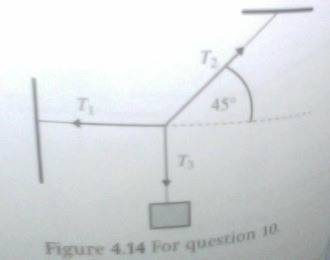# Find the tension in each string

## Homework Statement

A mass of 5.00 kg hangs attached to three strings as shown in the figure (see image below). Find the tension in each string. Hint: Consider the equilibrium of the point where the strings join.

W=mg

## The Attempt at a Solution

Well finding the tension in string 3 (T3) was relatively easy:

w=mg
mass of the block = 5.00 kg
g = 10 ms-2
w = 5.00 x 10 = 50 N

It is the other two (T1 and T2) i'm not sure. In fact I'm at lost with what I learned in class and applying. I noticed the problem states a hint (equilibrium where strings join) but I'm not seeing how this information can be used.

http://postimage.org/image/63m5xydbj/

SammyS
Staff Emeritus
Homework Helper
Gold Member

## Homework Statement

A mass of 5.00 kg hangs attached to three strings as shown in the figure (see image below). Find the tension in each string. Hint: Consider the equilibrium of the point where the strings join.

W=mg

## The Attempt at a Solution

Well finding the tension in string 3 (T3) was relatively easy:

w=mg
mass of the block = 5.00 kg
g = 10 ms-2
w = 5.00 x 10 = 50 N

It is the other two (T1 and T2) i'm not sure. In fact I'm at lost with what I learned in class and applying. I noticed the problem states a hint (equilibrium where strings join) but I'm not seeing how this information can be used.

[PLAIN]http://postimage.org/image/63m5xydbj/[/QUOTE] [Broken]
First, I'll display the image, although it's of a fairly poor quality.Draw a free body diagram for the point at which the three strings meet.

.

#### Attachments

Last edited by a moderator:
Thank you Sammy. I noticed what I had done wrong, I ignored to look at the vectorial components. After that, the rest unfolded easily. Thanks again :)